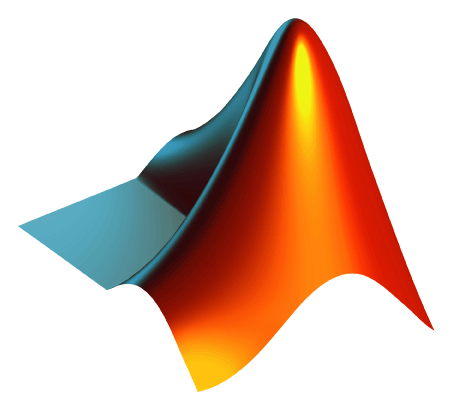Community Profile# Jos Martin

### MathWorks

Last seen: 16 days ago Active since 2011

Director Of Engineering - Cloud Integration and Parallel Computing.#### Statistics

All
•••••••#### Content Feed

View by

Channel

BBH Internal Network

1 year ago

Channel

router-01-external-ping

2 years ago

Channel

router-01

2 years ago

Solved

Finding Perfect Squares
Given a vector of numbers, return true if one of the numbers is a square of one of the other numbers. Otherwise return false. E...

3 years ago

Solved

Piecewise linear interpolation
Given an Mx2 vector and a row of M-1 integers, output a two column vector that linearly interpolates Y times between each succes...

4 years ago

Solved

How to subtract?
*&plusmn &plusmn &plusmn &plusmn &plusmn &plusmn &plusmn &plusmn &plusmn &plusmn &plusmn* * Imagine you need to subtract one...

5 years ago

Submitted

Port Passing Problem
Monte-carlo and analytic investigation of a random walk processSubmitted

Hydrogenic Wavefunction Visulization Tool
Visualize hydrogenic wave-functions in 3D and watch animations of atomic state changesSubmitted

Cluster setup scripts
Shell scripts to easily control a MATLAB distributing computing cluster

Submitted

Visual Timing Report for Distributed Tasks
Create a visual representation of when and where each task in a distributed computing job executes.Solved

Is my wife right?
Regardless of input, output the string 'yes'.

7 years ago

Channel

Burwell Router

7 years ago

Channel

CurlTest

7 years ago

Channel

7 years ago

Solved

Select every other element of a vector
Write a function which returns every other element of the vector passed in. That is, it returns the all odd-numbered elements, s...

10 years ago

GPU computing with OS X 10.8 and retina macbook
We believe that the hang you are seeing relates to the CUDA driver recompiling all of the GPU libraries to run on the particular...

10 years ago | 4

Matlab not recognizing cuda driver 5
There appears to be an interaction between "automatic graphics switching" in Mountain Lion and the underlying NVIDIA drivers. Co...

10 years ago | 0

Solved

Function composition - harder
Write a function that accepts an arbitrary number of function handles f_1, f_2, ..., f_n and returns the composition h. That is,...

11 years ago

Solved

Given a and b, return the sum a+b in c.

11 years ago

Solved

Determine if input is odd
Given the input n, return true if n is odd or false if n is even.

11 years ago

Solved

Make a checkerboard matrix
Given an integer n, make an n-by-n matrix made up of alternating ones and zeros as shown below. The a(1,1) should be 1. Example...

11 years ago

Solved

Find the sum of all the numbers of the input vector
Find the sum of all the numbers of the input vector x. Examples: Input x = [1 2 3 5] Output y is 11 Input x ...

11 years ago

Solved

Make the vector [1 2 3 4 5 6 7 8 9 10]
In MATLAB, you create a vector by enclosing the elements in square brackets like so: x = [1 2 3 4] Commas are optional, s...

11 years ago

Solved

Times 2 - START HERE
Try out this test problem first. Given the variable x as your input, multiply it by two and put the result in y. Examples:...

11 years ago